## Tamilnadu Samacheer Kalvi 9th Maths Solutions Chapter 5 Coordinate Geometry Ex 5.6

Multiple Choice Questions :

Question 1.
If the y-coordinate of a point is zero, then the point always lies
(3) on x-axis
(4) on y-axis
Solution:
(3) on x-axis

Question 2.
The points (-5, 2) and (2, -5) lie in the ___.
(2) II and III quadrant respectively
(3) II and IV quadrant respectively
(4) IV and II quadrant respectively
Hint: (-, +) lies IInd quadrant and (+, -) lies in IVth quadrant
Solution:
(3) II and IV quadrant respectively

Question 3.
On plotting the points 0(0, 0), A(3, – 4), B(3, 4) and C(0, 4) and joining OA, AB, BC and CO, which of the following figure is obtained?
(1) Square
(2) Rectangle
(3) Trapezium
(4) Rhombus
Hint: In trapezium one pair of opposite side is parallel.
Solution:
(3) TrapeziumQuestion 4.
If P(-1, 1), Q(3, -4), R(1, -1), S(-2, -3) and T(-4, 4) are plotted on a graph paper, then the points in the fourth quadrant are ____.
(1) P and T
(2) Q and R
(3) only S
(4) P and Q
Hint: Points in IVth quadrant are (+, -)
Solution:
(2) Q and R

Question 5.
The point whose ordinate is 4 and which lies on they-axis is
(1) (4, 0)
(2) (0, 4)
(3) (1, 4)
(4) (4, 2)
Hint: Points in y-axis have abscissa a zero
Solution:
(2) (0, 4)

Question 6.
The distance between the two points (2, 3) and (1, 4) is ___ .
(1) 2
(2) $$\sqrt{56}$$
(3) $$\sqrt{10}$$
(4) $$\sqrt{2}$$
Hint: Distance = $$\sqrt{\left(x_{2}-x_{1}\right)^{2}+\left(y_{2}-y_{1}\right)^{2}}$$
Solution:
(4) $$\sqrt{2}$$

Question 7.
If the points A (2, 0), B (-6, 0), C (3, a – 3) lie on the x-axis then the value of a is ___.
(1) 0
(2) 2
(3) 3
(4) -6
Hint: Points in y-axis have ordinate zero. ∴ a – 3 = 0 ⇒ a = 3
Solution:
(3) 3

Question 8.
If ( x + 2, 4) = (5, y – 2), then the coordinates (x, y) are ___.
(1) (7, 12)
(2) (6, 3)
(3) (3, 6)
(4) (2, 1)
Hint: x + 2 = 5 ⇒ x = 3; y – 2 = 4 ⇒ y = 6
Solution:
(3) (3, 6)Question 9.
If Q1, Q2, Q3, Q4 are the quadrants in a Cartesian plane then Q2 ∩ Q3 is
(1) Q1 ∪ Q2
(2) Q2 ∪ Q3
(3) Null set
(4) Negative x-axis
Hint: Quadrants do not contain the axis.
Solution:
(3) Null set

Question 10.
The distance between the point (5, -1) and the origin is
(1) $$\sqrt{24}$$
(2) $$\sqrt{37}$$
(3) $$\sqrt{26}$$
(4) $$\sqrt{17}$$
Hint: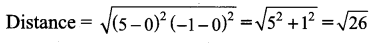Solution:
(3) $$\sqrt{26}$$

Question 11.
The coordinates of the point C dividing the line segment joining the points P(2, 4) and Q(5, 7) internally in the ratio 2 : 1 is
(1) $$\left(\frac{7}{2}, \frac{11}{2}\right)$$
(2) (3, 5)
(3) (4, 4)
(4) (4, 6)
Hint: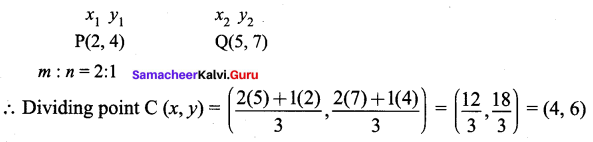Question 12.
If P$$\left(\frac{a}{3}, \frac{b}{2}\right)$$ is the mid-point of the line segment joining A(-4, 3) and B(-2, 4) then (a, b)
(1) (-9, 7)
(2) $$\left(-3, \frac{7}{2}\right)$$
(3) (9, -7)
(4) $$\left(3-\frac{7}{2}\right)$$
Hint: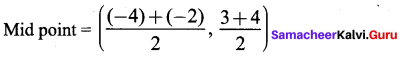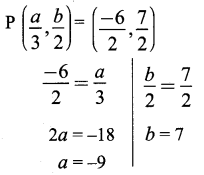Solution:
(1) (-9, 7)

Question 13.
In what ratio does the point Q(1, 6) divide the line segment joining the points P(2, 7) and R(-2, 3)
(1) 1 : 2
(2) 2 : 1
(3) 1 : 3
(4) 3 : 1.
Hint: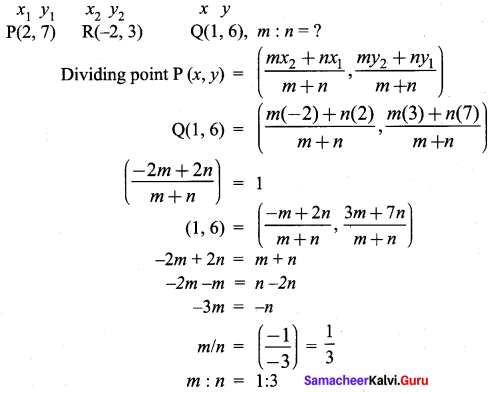Question 14.
If the coordinates of one end of a diameter of a circle is (3, 4) and the coordinates of its center is (-3, 2), then the coordinate of the other end of the diameter is
(1) (0, -3)
(2) (0, 9)
(3) (3, 0)
(4) (-9, 0)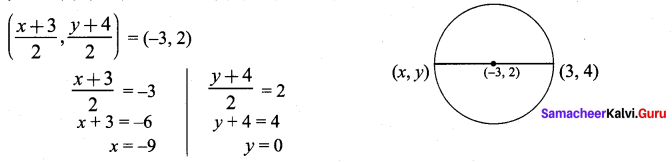Solution:
(4) (-9, 0)

Question 15.
The ratio in which the x-axis divides the line segment joining the points A(a1, b1) and B(a2, b2) is
(1) b1 : b2
(2 ) -b1 : b2
(3 ) a1 : a2
(4 ) -a1 : a2
Hint: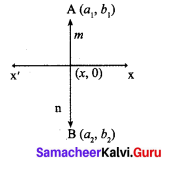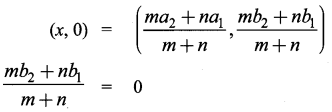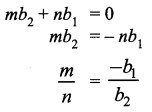Solution:
(2 ) -b1 : b2

Question 16.
The ratio in which the x-axis divides the line segment joining the points (6, 4) and (1, -7) is
(1) 2 : 3
(2) 3 : 4
(3) 4 : 7
(4) 4 : 3
Hint: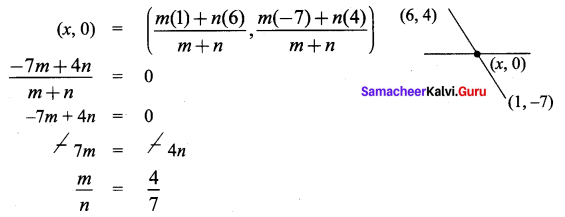Solution:
(3) 4 : 7

Question 17.
If the coordinates of the mid-points of the sides AB, BC and CA of a triangle are (3, 4), (1, 1) and (2, -3) respectively, then the vertices A and B of the triangle are
(1) (3, 2), (2, 4)
(2) (4, 0), (2, 8)
(3) (3, 4), (2, 0)
(4) (4, 3), (2, 4)
Hint: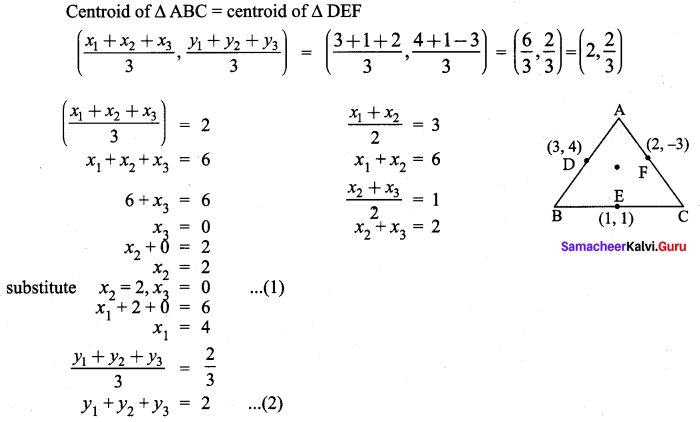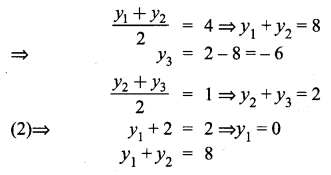0 + y2 = 8 ⇒ y2 = 8
(x1, y1) = (4, 0) ; (x2, y2) = (2, 8)
Solution:
(2) (4, 0), (2, 8)

Question 18.
The mid-point of the line joining (-a, 2b) and (-3a, -4b) is
(1) (2a, 3b)
(2) (-2a, -b)
(3) (2a, b)
(4) (-2a, -3b)
Hint: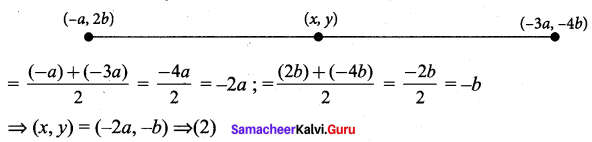Solution:
(2) (-2a, -b)

Question 19.
In what ratio does the j-axis divides the line joining the points (-5, 1) and (2, 3) internally
(1) 1 : 3
(2) 2 : 5
(3) 3 : 1
(4) 5 : 2
Hint: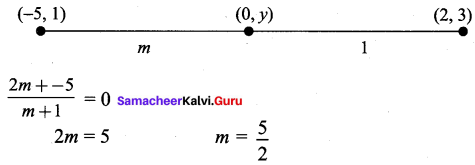Solution:
(4) 5 : 2

Question 20.
If (1, -2), (3, 6), (x, 10) and (3, 2) are the vertices of the parallelogram taken in order, then the value of x is
(1) 6
(2) 5
(3) 4
(4) 3
Hint:
In parallelogram diagonals bisect each other.
Mid point of AC = Mid point of BD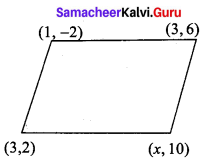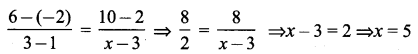Solution:
(2) 5